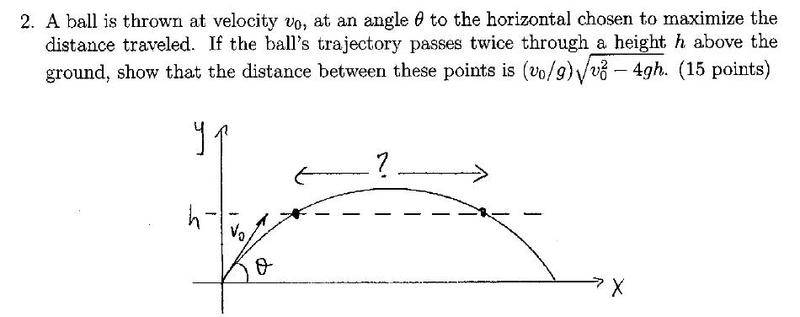# Distance between 2 points on a projectile's path

aftershock

## Homework Statement

This doesn't seem like much more than a kinematics problem but it's for an upper division mechanics course so I chose to post it here. Feel free to move to intro if necessary.## Homework Equations

kinematics equations

## The Attempt at a Solution

I focused only on the vertical component to find the speed at point 1:

V2=Vy2 -2gh

V=√(Vy2 -2gh)

Now I found the time it takes to get from point 1 to the top of the path:

0 = √(Vy2 -2gh) -gt

t = √(Vy2 -2gh) /g

To get from the top to point 2 would take the same amount of time so the total time to get from point 1 to 2 is 2*√(
Vy2 -2gh) /g

Since there's no acceleration in the x direction the distance between the two points is

2Vx*√(Vy2 -2gh) /g

I think this is the correct answer, however I can't get it to match the given solution. Furthermore even if this is correct it seems like it would take a lot of algebra to match the given solution, leading me to believe another approach was taken. Any help?

voko
There was another condition: the distance traveled must be maximized. That means a particular condition on Vx and Vy. Get it, then plug into your equation.

aftershock
There was another condition: the distance traveled must be maximized. That means a particular condition on Vx and Vy. Get it, then plug into your equation.

As in theta should be 45 degrees?

EDIT: This would mean the horizontal and vertical components are initially equal making the equations match. Thank you!

Last edited:
Oxvillian
Try looking at it this way:

You're probably effectively solving the quadratic equation y=h. The distance between the two solutions is going to be twice the discriminant appearing in your quadratic formula.

Mentor
Express Vx & Vy in terms of V0. (Use the information in the problem statement.)

Edit: voko beat me to it.